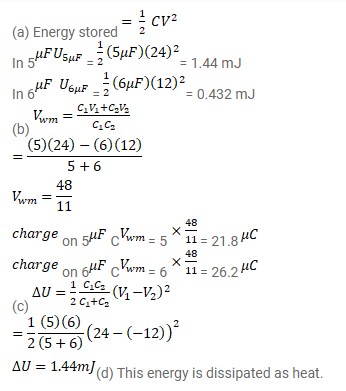# A capacitor of capacitance 5.00 μF is charged to 24.0 VQuestion:

A capacitor of capacitance $5.00 \mu \mathrm{F}$ is charged to $24.0 \mathrm{~V}$ and another capacitor of capacitance 6 ' $0 \mu \mathrm{F}$ is charged to $12.0 \mathrm{~V}$.

(a) Find the energy stored in each capacitor,

(b) The positive plate of the first capacitor is now connected to the negative plate of the second and vice versa. Find the. new charges on the capacitors,

(c) Find the loss of electrostatic energy during the process,

(d) Where does this energy go ?

Solution: